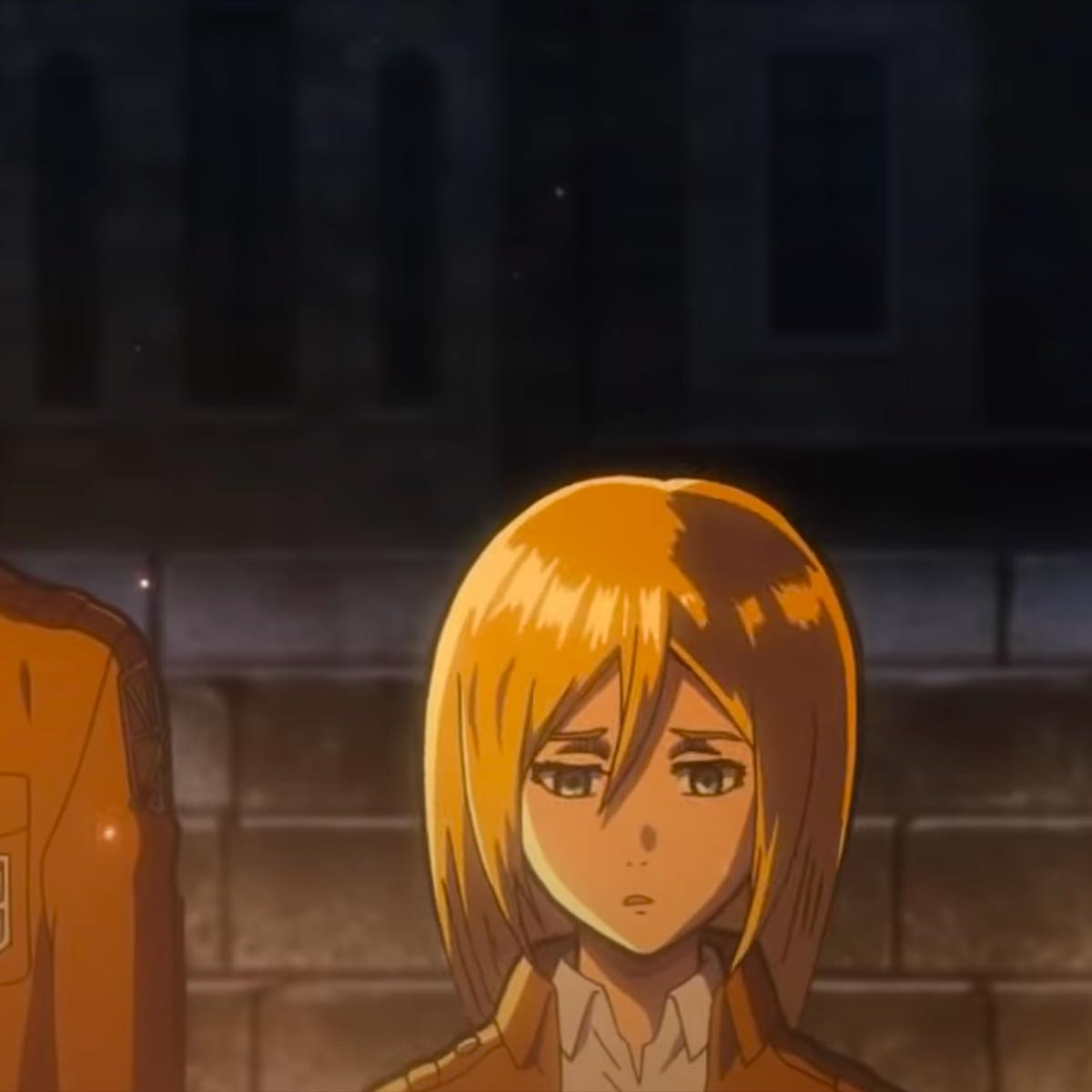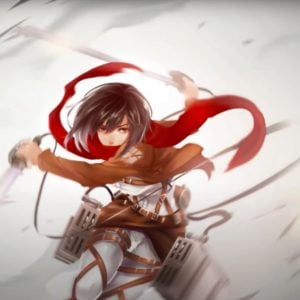# Jiyuu No Tsubasa (Attack on Titan)

10 September 2020
3
01:25
• ##### TEMPO
160
0
(0)

osdf f h h s|df| g f d s s|
aa osdf f h h s|df| g f d s
psasdsasdsasdsao
psasdsasdsasdsao
psasdsasdsasdsao
psasdsasdsasdsao
psasdsaoG|||
S|G|S f|dSa|pa| SdSII|S d
S|af d f| a f| H|
SSSd a S O Op a
SSSd a S O Op a
S|G|j H Gf|dS|
SSdSS|aa|
S|G|j k jH GGfGf| pasSSSd a a
S|G|j H Gf|dS| SSdfS|aa|
S|G|j k jH GGfGf| pasSSSd a a
S|G|j H|jjHGHH|GG| G| G|
G| f| G| f d| f| j| j|
j| h| j| h J| H| jjjJ h j

Rate This Music Sheet:

Average rating 0 / 5. Vote count: 0

No votes so far! Be the first to rate this music sheet.

Thank you for rating this song!

If you have any specific feedback about how to improve this music sheet, please submit this in the box below.

Jiyuu No Tsubasa (Attack on Titan) is a song by Linked Horizon. Use your computer keyboard to play Jiyuu No Tsubasa (Attack on Titan) music sheet on Virtual Piano. This is an Easy song and requires practice. The recommended time to play this music sheet is 01:25, as verified by Virtual Piano legend, Mark Chaimbers. The song Jiyuu No Tsubasa (Attack on Titan) is classified in the genres: Japan, Songs From TV, Manga on Virtual Piano. You can also find other similar songs using Anime, Attack On Titan.

## Other Songs By Linked Horizon

•[toD]| |D o D [yog]| |g o g [shY]| |h o h [siH]| |[oJD]| |[PJD%] ( W [PE] t|D g d [d^] q E t [yd] s s d D [o8] w t y [oY] P t Y [y5]| |d h Z [z8]|[dY]|o P z h c Z z| |d h Z [zg^J]|[yi]|o P d z h c Z z| |z Z c [vhW] Y O P [sl]|l l B V [vD] c [hZ] z Z c [zE] i [PJ] s [hd] g z h d D d s| |y o D [od8] w t Y i d o g [og] D d i|y o D [^D] d w E t y d o g g D d t y [ts] D J [PJ%] ( W E t P D H h E [tg] Y [iD]|D d D g [od^] q E t y i d i [d5] 9 w y [od] [YD] [yd] [YD] [igP%] ( W [ohE] [tD]||h D ^ q [dE] t y d d D [g8] w t [hY] [si]|o s 5 ( w E t D D d [pd\$] 9 Q e t D D d [dO4] 8 q W t d D g [D5] 7 [od9] w r y [ohda] w y o d|[oh] [ZD] [zd] [gc] [hZD1] 5 8 ( w [ZJD(] [zdE] ( [g^] J c w [ZED] t y [tZD] [zyd] [toh] [h%] l Z v ( [lgcW] E [tlgc] E [tZD] E [slh5] 9 [wPJ] 9 E [PJ9] [wOH] [oh9] [OHD4] 8 q [ZD8] [ZWD] 8 q [oh8] [OH5] 9 w [gcJ9] [gcJE] 9 [wZD] [gc9] [h%] l Z v [VH(] [vth] [gc(] E [ZD(] [zdE] [JB(] ^ q E q [oED] [qd] [ED] [qoh] [sg1] 5 [oD8] ( w [oD(] [wd] [h(] [og^] w [ED] t y [tsD] [yJ] [tsH] [sH%] [shO(] [shWO] [sgOE] [tsgO] [soED] [tsoD] [hPE] [og5] 9 [wod] 9 E 9 [wod]|[tqdW] D d o [ywd] D d o [sYW] o [dE] o [tD] o d|[yw84] Y y w [y95] Y y w [t%] @ [Y%] @ [wti^] 4 [ywo^] 4 [Y1] y [Y5] w [Y8] y [Y5] w [Y(] y [Y5] w [Y8] y [Y5] w [^Y] y [Y5] w [^Y] y [Y8] w [Y9] y [Y8] w [Y9] y [Y8] w [Y%] y [[email protected]] w [Y%] y [^Y] w [Y8] y [^Y] w [Y8] y [^Y] w [^Y] w [i4] w [o^] w [i4] w [Y8] w [y4] w [^Y] w [i4] w [o1] w [t5] o [w8] t [o5] w [o(] w [o5] w [t8] O [w5] w [o^] w [t5] o [w^] t [o5] w [t8] o [w5] ^ [o5] O [o%] w [[email protected]] w [Y%] w [y^] w [Y8] w [i^] 8 ^ [o^Y] [iO4] [o^P] [sO4] [d^P] [PD4] [gd^P] [hPD4] [tso1]| |m v v V c c v m m l s t 8

Level: 6
Length: 03:24
Intermediate
##### Omake Pfadlib (Attack on Titan)

•p| s| [qpf4]||| [w5]| |[ywoa]| s| [oed6]||| | |[utoe]| a| [q4]| [p8]| q| | [w5]| |[ywod]| |[soe6]||| | |p| s| [qpf4]||| [w5]| |[ywoa]| s| [oed6]| 0| [se]| | | |[utohe]| d| [q4]| [s8]| q| | [w5]| |[ywod]| | [soe6]| 0| e| t| u| |e| u| [uq4]| 8| q| u r [w5]| 9| w| e t [e6]| 0| e| 0| r| 0| e| u| [uq4]| 8| q| u a [w5]| 9| w| s [uo] [e6]| 0| e| 0| r| 0| [pe]| [f0]| [qpf4]| 8| q| f [oa] [w5]| 9| w| s [uo] [e6]| 0| e| 0| r| 0| [oe]| [u0] o [q4]| 8| q| 8| [w5]| 9| [wd]| [s9] d [e6]| 0| e| s [up] | 7| 0| r
Level: 7
Length: 01:12
Intermediate
##### Call of Silence (Attack on Titan)

•DDGg SSD DGg S|| J GH g G D g S J GH g G D g S L jk H j G H f L jk H j G H f IafjgsO g SD s S P s O g SD s S s P O g SD s S P s O g SD s S s P O g SD s S P s O g SD s S s P O g SD s S P s O g SD s S s P O P PPP sSsPp|ip P iPs isS iSD D DDDP D SSSO S D DDD P D D dd| D DGg SSD DGg S D DGg D D S P S D Gg SSD DGg S DDGg DS P J|H| D DGg SSD DGg S D DGg D D S P S D DGg S DDGg SD Gg DS D|| J GH g G D g S L jk H j G H f L jk H j G H f L jk H j G H f L jk H j G H f x lz k l j k h x lz kl||xvb
Level: 2
Length: 01:06
Easy

## Related

•[8s] f o|[0o]|
[qp] s i|[qi]|
[wu] o t|[5r]|
[8r]|5|[1t]|
[5w] r y|y|
[5w] t u|u|
5 w[7r]yi8 u[wu]|9|[5y]|
[8s] f o|[0o]|
[qp] s i|[qi]|
[wu] o t|[5r]|
[8r]|5|[1t]|
[5w] r y|y|
[5w] t u|u|
5 w[7r]yi8 u[wu]|9|[5y]|
[*o] P u|u|
[9i] p y|y|
[wu] o [eT]|[6T]|
[9T]|6|[2y]|
[7i] p y|y|
[8u] o t|t|
[qy] i [wr]|[5r]|
[8r]|5|[1t]|
[0s] f o|o|
[qp] s i|i|
[wu] o t|[5r]|[6r]||t|
[0s] f o|o|
[qp] s i|i|
[wu] o t|[5r]|
[8r]|5|[1t]

Level: 4
Length: 00:48
Easy
##### Minuet in F Major

•P D [igdP]| |D|[hYD]| |D|[iH]|[hY]|g|[hE]| |D|[oPJ]| |h|[tlY]| |h|[JE]|[iH]|h|[hYPE]| |E| |[igTE]|E| |E| |[w^P(]| |[PE]| |[q^P]| |P|J| |H|s|D| |d| |@ ^ ( q w E [qW]|[w(]| |5 9 w e E y [tW]|[wE]| |[ZD] Y D d s P O o O P s d [JD] Y D d s d D g h H J l J D [oZ]|P| |s|[PJ] S L| |S| |[lO]|H| |[YD]| |P|g|D|[[email protected]] ^ w|[h(] h [H9] ^ [h9] W [wg] q [h(] [s8] [D(] 8 ( [h^] [H(] 8 [s(] 8 [d(] q @ ^ [wh] ^ ( [h^] [l9] ^ [J9] [WJ] [wD] q [wh] [s(] [WD] ( E [h(] [tH] ( [s8] ( [dW] E t W [tJ] W t W y q [zy] q [yJ] q [JE] q E q E [qJ] [h(] 8 [qH] [H8] [wJ] [J8] [WJ] [D(] W [J(] [WJ] [l(] [WJ] [D(] w [P(] [qg] [D(] [qg] ^ [h(] ^ w ^ [h(] ^ [qH] ^ [wJ] ^ t W [tZ] W t W y q [yc] q [zy] q [JE] q E t [yc] [ic] [cY] E [iZ] E o [hE] [WH] % ( [WH] % ( [D(] % ( w q [D(] [D(] % 8 ( [D%] [D8] [z9]| |E| |P D [igdP]| |D|[hYD]| |[iH]|[hY]|g|[hE]| |[hE]| |[wh]|[tl]| |[hE]|[oJ]|[iH]|h h [hE]| |E| |h|[gTE]| |^| |[tW]| |[wE]| |D|[qWD]| |E

Level: 5
Length: 02:43
Intermediate

#### Masaru Yokoyama

•p|fDfDfDfs p|psfg s g h fgfgf x p|fDfDfDfs p|psfg s g h [uf][ig][uf][ig][uf] [xf] p|fDfDfDfs p|psfg s h g fgfgf x
Level: 4
Length: 00:20
Easy